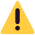## ​سافت موبایل ، خانه ی تعمیرکاران نرم افزار موبایل# کردیت نرم افزار EMT Standard Edition

کد محصول: 345074
۳۹,۹۰۰ تومان

جهت فعال سازی کردیت،یوزرنیمی که قبلا با آن در سایت emegsm.com رجیستر کرده اید را کادر زیر برای ما ارسال نمایید.

حداقل تعداد کردیت جهت خرید: 7 عدد

افزودن به سبد خرید
• مشخصات محصول
• بررسی
• فعال سازی
• قابلیت
• نظرات
•## با عرض پوزش خدمت کاربران عزیز:فروش تا سه شنبه مورخ 1401/2/27 بسته می باشد.

سبد خرید
• <%- item.title %>
<% if(item.model) { %>
<%- item.model %>
<% } %>
تعداد:
<% if(item.compare_price) { %> <%- ((item.compare_price.toString().formatNumber().convertToLocalNumber()) + currency_sign) %> <% } %> <%- ((item.price.toString().formatNumber().convertToLocalNumber()) + currency_sign) %>
• رمز عبورتان را فراموش کرده‌اید؟

ثبت کلمه عبور خود را فراموش کرده‌اید؟ لطفا شماره همراه یا آدرس ایمیل خودتان را وارد کنید. شما به زودی یک ایمیل یا اس ام اس برای ایجاد کلمه عبور جدید، دریافت خواهید کرد.

بازگشت به بخش ورود

کد دریافتی را وارد نمایید.

بازگشت به بخش ورود

### مشاهده سفارش

<%- order.customer_name.toString() %>
<%- order.id.toString().convertToLocalNumber() %>
<%- order.customer_province.toString() %>-<%- order.customer_city.toString() %>-<%- order.customer_address.toString() %>
<%- order.customer_mobile.toString().convertToLocalNumber() %>
<%- order.shipping_name.toString() %>
<%- (Number(order.total_shipping).toString().formatNumber().convertToLocalNumber() + currency_sign) %>
<%- order.payment_method_name.toString() %>
<%- (Number(order.total).toString().formatNumber().convertToLocalNumber() + currency_sign) %>
<% if(order.tracking_number) { %>
<%- order.tracking_number.toString() %>
<% } %>
نام محصول
تعداد
قیمت واحد
قیمت کل
تخفیف
قیمت نهایی
<% \$.each(products, function(index,product) { %><%- product.name %>
<%- product.quantity %>
<%- (Number(product.original_price).toString().formatNumber().convertToLocalNumber() + currency_sign) %>
<%- (Number(product.original_price*product.quantity).toString().formatNumber().convertToLocalNumber() + currency_sign) %>
<%- (Number(product.discount).toString().formatNumber().convertToLocalNumber() + currency_sign) %>
<%- (Number(product.total).toString().formatNumber().convertToLocalNumber() + currency_sign) %>
<% }); %>
<% if(!orders.length) { %>

شما هنوز هیچ سفارشی ثبت نکرده‌اید.

<% } else { %>
• شماره سفارش
تاریخ سفارش
پرداخت
وضعیت
جمع نهایی
• <% \$.each(orders, function(index,order) { %>
• <%- order.id.toString().convertToLocalNumber() %>